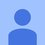# Systems of Equations by Using Elimination Method

**Take Note** Systems of Equation- 2 or more equations with the same set of variables

Solving Systems of Equations 1) Substitution method 2)Elimination Method 3) Graphing Method **Take Note**

Substitution Method Steps: a. Solve for x an y of one equation b. Substitute the answer of step a to the other equation and solve further for the other variable c. Substitute the answer of step b to the equation used in step a

Examples: Given: 2x+y=-3 2x-y=-5 a. to find the y, we should first isolate it. To do that, we will transpose any variable with it which 2x. (I chose the first equation to solve.) This would be the equation after transposing it: y=-2x-3 Which is the end of step a.

b. we will substitute the equation of y to the second equation which is from 2x-y=-5 to 2x-(-2x-3)=-5. Distribute the y to the equation...Negative multiplied to another negative? Positive!! So from 2x-(-2x-3)=-5, simplify, is equal to 2x+2x+3=-5. Simply it again to 4x+3=-5. Now, we isolate the x. Transpose 3 to the other side 4x=-5-3 to 4x=-8. Divide both sides by 4 (since the variable x has a constant of 4, in order to remove the 4, we divide it to both sides ;) ) Now its now x=-2 :D Which is the end of step b

c. So now we replace the x in the y's equation which is y=-2x-3 To y=-2(-2)-3. Simplify, y=4-3 to y=1. End of the last step, step c. :D

To check if its correct, just replace both final answers to ANY equation. So, using the first equation, 2x+y=-3 Substitute, 2(-2)+1=3 -4+1+3 3=3

Easy Peasy right? Hahahaha hope this helps! :DNote by Chirito Kazuya
6 years, 7 months ago

This discussion board is a place to discuss our Daily Challenges and the math and science related to those challenges. Explanations are more than just a solution — they should explain the steps and thinking strategies that you used to obtain the solution. Comments should further the discussion of math and science.

When posting on Brilliant:

• Use the emojis to react to an explanation, whether you're congratulating a job well done , or just really confused .
• Ask specific questions about the challenge or the steps in somebody's explanation. Well-posed questions can add a lot to the discussion, but posting "I don't understand!" doesn't help anyone.
• Try to contribute something new to the discussion, whether it is an extension, generalization or other idea related to the challenge.

MarkdownAppears as
*italics* or _italics_ italics
**bold** or __bold__ bold
- bulleted- list
• bulleted
• list
1. numbered2. list
1. numbered
2. list
Note: you must add a full line of space before and after lists for them to show up correctly
paragraph 1paragraph 2

paragraph 1

paragraph 2

[example link](https://brilliant.org)example link
> This is a quote
This is a quote
    # I indented these lines
# 4 spaces, and now they show
# up as a code block.

print "hello world"
# I indented these lines
# 4 spaces, and now they show
# up as a code block.

print "hello world"
MathAppears as
Remember to wrap math in $$ ... $$ or $ ... $ to ensure proper formatting.
2 \times 3 $2 \times 3$
2^{34} $2^{34}$
a_{i-1} $a_{i-1}$
\frac{2}{3} $\frac{2}{3}$
\sqrt{2} $\sqrt{2}$
\sum_{i=1}^3 $\sum_{i=1}^3$
\sin \theta $\sin \theta$
\boxed{123} $\boxed{123}$